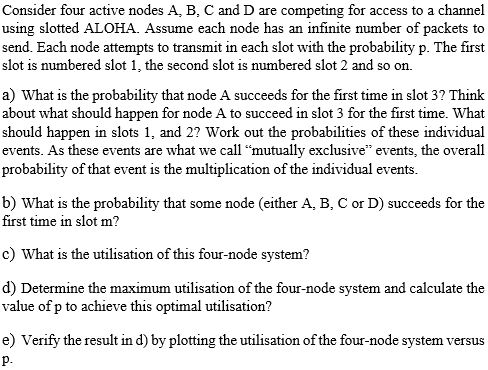Home / Expert Answers / Electrical Engineering / consider-four-active-nodes-a-b-c-and-d-are-competing-for-access-to-a-channel-using-slotted-aloha-pa661

# (Solved): Consider four active nodes A, B, C and D are competing for access to a channel using slotted ALOHA ...Consider four active nodes A, B, C and D are competing for access to a channel using slotted ALOHA. Assume each node has an infinite number of packets to send. Each node attempts to transmit in each slot with the probability p. The first slot is numbered slot 1 , the second slot is numbered slot 2 and so on. a) What is the probability that node A succeeds for the first time in slot 3? Think about what should happen for node A to succeed in slot 3 for the first time. What should happen in slots 1 , and 2 ? Work out the probabilities of these individual events. As these events are what we call "mutually exclusive" events, the overall probability of that event is the multiplication of the individual events. b) What is the probability that some node (either A, B, C or D) succeeds for the first time in slot ? c) What is the utilisation of this four-node system? d) Determine the maximum utilisation of the four-node system and calculate the value of to achieve this optimal utilisation? e) Verify the result in d) by plotting the utilisation of the four-node system versus p.

We have an Answer from Expert